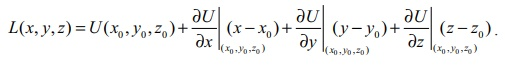Home | | Maths 12th Std | Linear Approximation and Differential of a function of several variables

# Linear Approximation and Differential of a function of several variables

Here we introduce similar ideas for functions of two variables and three variables. In general for functions of several variables these concepts can be defined similarly.

Linear Approximation and Differential of a function of several variables

Earlier in this chapter, we have seen that linear approximation and differential of a function of one variable. Here we introduce similar ideas for functions of two variables and three variables. In general for functions of several variables these concepts can be defined similarly.

### Definition 8.10

Let A = {( x, y ) | a < x < b, c < y < d } ŌŖé ŌäØ2 , F : A ŌåÆ ŌäØ, and ( x0 , y0 ) Ōłł A .

(i) The linear approximation of F at ( x0 , y0 ) Ōłł A is defined to be(ii) The differential of F is defined to bewhere dx = ╬öx and dy = ╬öy,

Here we shall outline the linear approximations and differential for the functions of three variables. Actually, we can define linear approximations and differential for real valued function having more variables, but we restrict ourselves to only three variables.

### Definition 8.11

Let A = {( x, y , z) | a < x < b, c < y < d, e < z < f } ŌŖé ŌäØ3, F : A ŌåÆ ŌäØ and ( x0 , y0 , z0 ) Ōłł A .

(i) The linear approximation of F at ( x0 , y0 , z0 ) Ōłł A is defined to be(ii) The differential of F is defined bywhere dx = ╬öx , dy = ╬öy and dz = ╬öz ,

Geometrically, in the case of function f of one variable, the linear approximation at a point x0 represents the tangent line to the graph of y = f ( x) at x0 . Similarly, in the case of a function F of two variables, the linear approximation at a point ( x0 , y0 ) represents the tangent plane to the graph of z = F ( x , y) at ( x0 , y0 ) .Example 8.16

If w( x, y , z) = x2y + y2z + z2x, x , y, z Ōłł ŌäØ, find the differential dw.

Solution

First let us find wx , wy , and wz .

Now wx = 2xy + z2, wy = 2yz + x2 and wZ = 2zx + y2.

Thus,by (15), the differential is

dw = (2xy + z2 )dx + (2 yz + x2 )dy + (2zx + y2)dz .

Example 8.17

Let U ( x, y , z) = x 2 ŌłÆ xy + 3sin z, x , y, z Ōłł ŌäØ. Find the linear approximation for U at (2, ŌłÆ1, 0) .

Solution

By (14), linear approximation is given byNow Ux = 2x ŌłÆ y , Uy = ŌłÆ x and U z = 3cos z .

Here (x0 , y0 , z0 ) = (2, ŌłÆ1, 0) , hence Ux (2, ŌłÆ1, 0) = 5, Uy (2, ŌłÆ1, 0) = ŌłÆ2 and Uz (2, ŌłÆ1, 0) = 3 .

Thus L ( x, y , z) = 6 + 5(x ŌłÆ 2) ŌłÆ 2( y + 1) + 3(z ŌłÆ 0) = 5x ŌłÆ 2 y + 3z ŌłÆ 6 is the required

Linear approximation for U at (2, ŌłÆ1, 0) .

Tags : Mathematics , 12th Maths : UNIT 8 : Differentials and Partial Derivatives
Study Material, Lecturing Notes, Assignment, Reference, Wiki description explanation, brief detail
12th Maths : UNIT 8 : Differentials and Partial Derivatives : Linear Approximation and Differential of a function of several variables | Mathematics